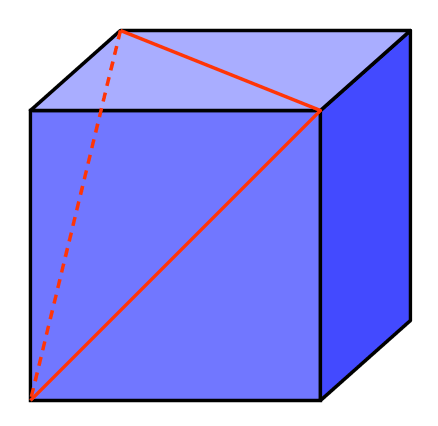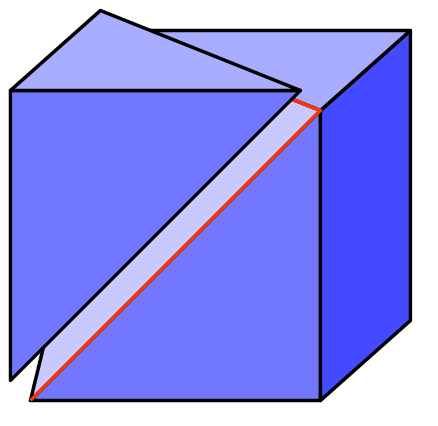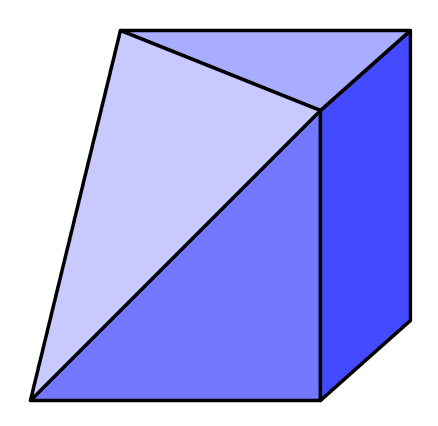GMAT Question of the Day: Daily via email | Daily via Instagram New to GMAT Club? Watch this Video

 It is currently 28 May 2020, 23:24### GMAT Club Daily Prep

#### Thank you for using the timer - this advanced tool can estimate your performance and suggest more practice questions. We have subscribed you to Daily Prep Questions via email.

Customized
for You

we will pick new questions that match your level based on your Timer History

Track

every week, we’ll send you an estimated GMAT score based on your performance

Practice
Pays

we will pick new questions that match your level based on your Timer History

#### Not interested in getting valuable practice questions and articles delivered to your email? No problem, unsubscribe here.# Question of the week - 46(A pastry is in the shape of a cube, .....)

Author Message
TAGS:

### Hide Tags

e-GMAT RepresentativeV
Joined: 04 Jan 2015
Posts: 3367
Question of the week - 46(A pastry is in the shape of a cube, .....)  [#permalink]

### Show Tags

1
300:00

Difficulty:75% (hard)

Question Stats:53% (02:40) correct48% (02:05) wrongbased on 80 sessions

### HideShow timer Statistics

Question of the Week - 46

A hollow box is in the shape of a cube, that has a side length of 2 metres. If a portion of it is cut along three adjacent face diagonals of the cube, then what is the total surface area of remaining larger piece of the box?

A. 4
B. 6
C. 16
D. 18
E. 24

_________________

Originally posted by EgmatQuantExpert on 28 Jun 2019, 02:33.
Last edited by EgmatQuantExpert on 30 Jun 2019, 17:17, edited 2 times in total.
GMAT Club LegendV
Joined: 18 Aug 2017
Posts: 6277
Location: India
Concentration: Sustainability, Marketing
GPA: 4
WE: Marketing (Energy and Utilities)
Re: Question of the week - 46(A pastry is in the shape of a cube, .....)  [#permalink]

### Show Tags

giving a try
visualize that the cut of the cake along three sides is done on one of the faces along the digaonal

TSA; 6*s^2 ; 24
and digaonal ; 2√2
along the diagonal cake is cut gives us three ∆ ; area;; 1/2 * 2*2 ; 2 *3 ; 6
left area l 24-6 = 18
IMO D

EgmatQuantExpert wrote:
Question of the Week - 46

A pastry is in the shape of a cube, that has a side length of 2 metres. If a portion of it is cut along three adjacent face diagonals of the cube, then what is the area of remaining larger piece of the pastry?

A. 4
B. 6
C. 16
D. 18
E. 24

Originally posted by Archit3110 on 28 Jun 2019, 09:50.
Last edited by Archit3110 on 29 Jun 2019, 02:28, edited 1 time in total.
DS Forum ModeratorV
Joined: 19 Oct 2018
Posts: 1845
Location: India
Re: Question of the week - 46(A pastry is in the shape of a cube, .....)  [#permalink]

### Show Tags

We will get smaller piece, a tetrahedron having 3 faces as right angle isosceles triangles whose equal sides are 2 cm, and fourth face is equilateral triangle having side $$2\sqrt{2}$$.
We can't find the area of a 3-D figure. It should be either volume, curved surface area or total surface area.

EgmatQuantExpert wrote:
Question of the Week - 46

A pastry is in the shape of a cube, that has a side length of 2 metres. If a portion of it is cut along three adjacent face diagonals of the cube, then what is the area of remaining larger piece of the pastry?

A. 4
B. 6
C. 16
D. 18
E. 24

Originally posted by nick1816 on 28 Jun 2019, 22:57.
Last edited by nick1816 on 29 Jun 2019, 18:12, edited 1 time in total.
InternB
Joined: 08 Apr 2019
Posts: 25
Location: India
GMAT 1: 700 Q50 V34
Re: Question of the week - 46(A pastry is in the shape of a cube, .....)  [#permalink]

### Show Tags

Archit3110 wrote:
giving a try
visualize that the cut of the cake along three sides is done on one of the faces along the digaonal

area = 2^3 ; 8
and digaonal ; 2√2
let three pieces be cut such that each has length ; √2 so we get two ∆ √2:√2:2
area of ∆ ; 1/2 * √2*√2 ; 1 since two ∆ are there so 1*2 ; 2
left area of pastry cube; 8-2 ; 6
IMO B

EgmatQuantExpert wrote:
Question of the Week - 46

A pastry is in the shape of a cube, that has a side length of 2 metres. If a portion of it is cut along three adjacent face diagonals of the cube, then what is the area of remaining larger piece of the pastry?

A. 4
B. 6
C. 16
D. 18
E. 24

Posted from my mobile device
CEOV
Joined: 03 Jun 2019
Posts: 2899
Location: India
GMAT 1: 690 Q50 V34WE: Engineering (Transportation)
Re: Question of the week - 46(A pastry is in the shape of a cube, .....)  [#permalink]

### Show Tags

2
1
EgmatQuantExpert wrote:
Question of the Week - 46

A pastry is in the shape of a cube, that has a side length of 2 metres. If a portion of it is cut along three adjacent face diagonals of the cube, then what is the area of remaining larger piece of the pastry?

A. 4
B. 6
C. 16
D. 18
E. 24

[url=https://e-gmat.com/quant-workshop-free-session/?
utm_source=GC&utm_medium=questions_q&utm_campaign=free_session&utm_content=quant_workshop&utm_term=GCquestions_q_fs_qow_qw][/url]

Total surface area of original cube = 6 * 4 = 24 sq m
Cut area = 3 * 1/2 *(2*2) = 6 sq m
Since there are 3 right angled triangles of base 2 & height 2 m cut from the original cube
Thus remaining area = 24 - 6 = 18 sq m

Alternatively remaining area = 3 * (2*2) + 3 * 1/2 (2*2) = 18 sq m
since there are 3 sides 0f 2*2 sq m = 12 sq m
and there are 3 right angles triangles of are = 3 * 1/2 (2*2) = 6 sq m

The solutions do not include area of equilateral triangle generated as a result of the cut = 2$$\sqrt{3}$$.
AttachmentsIMG_1402.jpg [ 500.91 KiB | Viewed 1296 times ]

_________________
Kinshook Chaturvedi
Email: kinshook.chaturvedi@gmail.com
InternB
Joined: 08 Apr 2019
Posts: 25
Location: India
GMAT 1: 700 Q50 V34
Re: Question of the week - 46(A pastry is in the shape of a cube, .....)  [#permalink]

### Show Tags

Kinshook wrote:
EgmatQuantExpert wrote:
Question of the Week - 46

A pastry is in the shape of a cube, that has a side length of 2 metres. If a portion of it is cut along three adjacent face diagonals of the cube, then what is the area of remaining larger piece of the pastry?

A. 4
B. 6
C. 16
D. 18
E. 24

[url=https://e-gmat.com/quant-workshop-free-session/?
utm_source=GC&utm_medium=questions_q&utm_campaign=free_session&utm_content=quant_workshop&utm_term=GCquestions_q_fs_qow_qw][/url]

Total surface area of original cube = 6 * 4 = 24 sq m
Cut area = 3 * 1/2 *(2*2) = 6 sq m
Since there are 3 right angled triangles of base 2 & height 2 m cut from the original cube
Thus remaining area = 24 - 6 = 18 sq m

Alternatively remaining area = 3 * (2*2) + 3 * 1/2 (2*2) = 18 sq m
since there are 3 sides 0f 2*2 sq m = 12 sq m
and there are 3 right angles triangles of are = 3 * 1/2 (2*2) = 6 sq m

The solutions do not include area of equilateral triangle generated as a result of the cut = 2$$\sqrt{3}$$.

Posted from my mobile device
e-GMAT RepresentativeV
Joined: 04 Jan 2015
Posts: 3367
Question of the week - 46(A pastry is in the shape of a cube, .....)  [#permalink]

### Show Tags

nick1816 wrote:
We will get smaller piece, a tetrahedron having 3 faces as right angle isosceles triangles whose equal sides are 2 cm, and fourth face is equilateral triangle having side $$2\sqrt{2}$$.
We can't find the area of a 3-D figure. It should be either volume, curved surface area or total surface area.

Hi @nikh1816,

When we say area, it implicitly means TSA. Anyway, we have edited the question to remove all the ambiguity.

Thanks & Regards,
_________________

Originally posted by EgmatQuantExpert on 30 Jun 2019, 06:49.
Last edited by EgmatQuantExpert on 30 Jun 2019, 17:21, edited 1 time in total.
GMAT Club LegendV
Joined: 18 Aug 2017
Posts: 6277
Location: India
Concentration: Sustainability, Marketing
GPA: 4
WE: Marketing (Energy and Utilities)
Re: Question of the week - 46(A pastry is in the shape of a cube, .....)  [#permalink]

### Show Tags

EgmatQuantExpert wrote:
nick1816 wrote:
We will get smaller piece, a tetrahedron having 3 faces as right angle isosceles triangles whose equal sides are 2 cm, and fourth face is equilateral triangle having side $$2\sqrt{2}$$.
We can't find the area of a 3-D figure. It should be either volume, curved surface area or total surface area.

Hi @nikh1816,

When we say area, it implicitly means TSA. Anyway, we have edited the question to remove all the ambiguity.

Thanks & Regards,
Sandeep.

EgmatQuantExpert ; thanks for editing the question .. else considering volume as answer would have been 6 ...
nick1816DS Forum ModeratorV
Joined: 19 Oct 2018
Posts: 1845
Location: India
Re: Question of the week - 46(A pastry is in the shape of a cube, .....)  [#permalink]

### Show Tags

Thanks for editing. But if we're considering TSA, then we should not exclude the area of the equilateral triangle that's left after we removed the cutting portion. None of the options is correct in that scenario. That's why i was confused. Imo question is poorly written and designed.

Archit3110 wrote:
EgmatQuantExpert wrote:
nick1816 wrote:
We will get smaller piece, a tetrahedron having 3 faces as right angle isosceles triangles whose equal sides are 2 cm, and fourth face is equilateral triangle having side $$2\sqrt{2}$$.
We can't find the area of a 3-D figure. It should be either volume, curved surface area or total surface area.

Hi @nikh1816,

When we say area, it implicitly means TSA. Anyway, we have edited the question to remove all the ambiguity.

Thanks & Regards,
Sandeep.

EgmatQuantExpert ; thanks for editing the question .. else considering volume as answer would have been 6 ...
nick1816e-GMAT RepresentativeV
Joined: 04 Jan 2015
Posts: 3367
Question of the week - 46(A pastry is in the shape of a cube, .....)  [#permalink]

### Show Tags

nick1816 wrote:
Thanks for editing. But if we're considering TSA, then we should not exclude the area of the equilateral triangle that's left after we removed the cutting portion. None of the options is correct in that scenario. That's why i was confused. Imo question is poorly written and designed.

Hi nick1816,

Thanks for pointing out the issue.

Apologies, we have edited the question now.

Thanks & Regards,
_________________
ManagerG
Joined: 29 Dec 2018
Posts: 71
Location: India
Re: Question of the week - 46(A pastry is in the shape of a cube, .....)  [#permalink]

### Show Tags

1
The question is still incorrect EgmatQuantExpert

Because the box is hollow, on cutting it along the given dimensions, the inside portion will get exposed and thus will increase the area. In fact, whatever is the surface area of the outside portion, the same area would be inside after cutting the hollow box.

Still, the question is wrong. Please check

Posted from my mobile device
ManagerS
Joined: 11 Feb 2018
Posts: 54
Re: Question of the week - 46(A pastry is in the shape of a cube, .....)  [#permalink]

### Show Tags

Archit3110 wrote:
giving a try
visualize that the cut of the cake along three sides is done on one of the faces along the digaonal

TSA; 6*s^2 ; 24
and digaonal ; 2√2
along the diagonal cake is cut gives us three ∆ ; area;; 1/2 * 2*2 ; 2 *3 ; 6
left area l 24-6 = 18
IMO D

EgmatQuantExpert wrote:
Question of the Week - 46

A pastry is in the shape of a cube, that has a side length of 2 metres. If a portion of it is cut along three adjacent face diagonals of the cube, then what is the area of remaining larger piece of the pastry?

A. 4
B. 6
C. 16
D. 18
E. 24

Hi Archit3110. Thanks for the solution. Could you please explain to me why it would be 3 triangles and not 4? what about the base of the shape is that not the 4th side. I followed the same method but when I tried to visualize the shape, to me the it seemed that the shape would have four faces. What am I missing?

e-GMAT RepresentativeV
Joined: 04 Jan 2015
Posts: 3367
Re: Question of the week - 46(A pastry is in the shape of a cube, .....)  [#permalink]

### Show Tags

Solution

Given:
• A hollow box is in the shape of a cube that has a side length of 2 meters
• A portion of it is cut along three adjacent face diagonals of the cube

To find:
• The total surface area of remaining larger piece of the box

Approach and Working out:
• A hollow box has all sides closed, and the total surface = 6 * area of a face.

Now, let us try to visualize the hollow box after it is cut along three adjacent face diagonals of the cube.• From the third figure, we can see that the total surface area of the larger piece = $$3 * area of a face + 3 * \frac{area of a face}{2}$$

Note: we should not consider the area of the triangle in total surface area, since the box is hollow.
• Area of a face = $$(side length)^2 = 2^2 = 4$$
• Therefore, the total surface area of the larger piece = $$3 * 4 + 3 * \frac{4}{2} = 12 + 6 = 18$$ sq. meters

Hence, the correct answer is Option D.

_________________
ManagerG
Joined: 29 Dec 2018
Posts: 97
Location: India
WE: Marketing (Real Estate)
Re: Question of the week - 46(A pastry is in the shape of a cube, .....)  [#permalink]

### Show Tags

EgmatQuantExpert wrote:

Solution

Given:
• A hollow box is in the shape of a cube that has a side length of 2 meters
• A portion of it is cut along three adjacent face diagonals of the cube

To find:
• The total surface area of remaining larger piece of the box

Approach and Working out:
• A hollow box has all sides closed, and the total surface = 6 * area of a face.

Now, let us try to visualize the hollow box after it is cut along three adjacent face diagonals of the cube.• From the third figure, we can see that the total surface area of the larger piece = $$3 * area of a face + 3 * \frac{area of a face}{2}$$

Note: we should not consider the area of the triangle in total surface area, since the box is hollow.
• Area of a face = $$(side length)^2 = 2^2 = 4$$
• Therefore, the total surface area of the larger piece = $$3 * 4 + 3 * \frac{4}{2} = 12 + 6 = 18$$ sq. meters

Hence, the correct answer is Option D.

This problem is so easy yet so difficult.

errr. Let me re-phrase my sentenceThis problem is so difficult if you cannot visualize the diagram, and so easy when you are able to visualize (or draw) it correctly. Thank you so much for the diagram EgmatQuantExpert_________________
Keep your eyes on the prize: 750Re: Question of the week - 46(A pastry is in the shape of a cube, .....)   [#permalink] 05 Aug 2019, 17:02

# Question of the week - 46(A pastry is in the shape of a cube, .....)